# What is the Definition for Congruent Line Segments for Geometry?

The term congruent in geometry means identical in shape and size. Congruent figures or objects have similar shape and size. In other words, if one object has the same shape and size as the mirror image of another object, then they are said to be congruent. There are many theorems related with congruency and equality. Two lines segments are said to be congruent if and only if they measure the same. Two angles are said to be congruent if and only if they measure the same. Two triangles are said to be congruent if and only if their corresponding parts are equal.

## Congruent definition in geometry

The word “congruent” means exactly similar in every feature of a figure in terms of shape and size. Even when the shapes are flipped or turned over, they remain congruent. Suppose two circles of the same radius are drawn on a piece of paper and cut them out and match up completely. Then we will see that they are of the same shape and size or it can be said that they are congruent. The symbol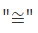is used to represent that one thing is congruent to another. If the angles of one shape is equal to another shape, then the angles are said to be congruent. Likewise, if the sides of one shape are equal in length to another shape, then the sides are congruent.

## What is Congruence?

The word congruence describes the relation of two figures which are congruent to each other. Congruent figures have similar shape and size whereas congruence fulfills many of the properties of equality. For example, two circles having equal radii are congruent. If two geometrical figures are congruent, they are mirror image to each other.

Let us look at the below given image.

If circle A is congruent to circle B, then it is written as: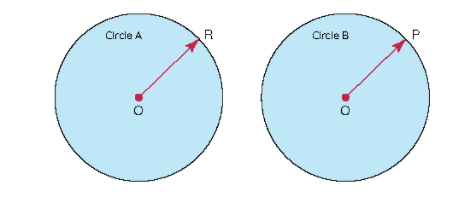## Properties of congruence

The following are the properties of congruence.

• Reflexive property- In congruence, reflexive property refers to a line segment or angle or shape which is congruent to itself. For example, for any angle P.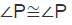• Symmetric property: In congruence, the symmetric property states that if one figure is congruent to another, then the second figure is also congruent to the first. For any two angles P and Q,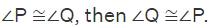• Transitive property: Transitive property of congruence mean that if two lines or angles or shapes are congruent to the third, then the first two lines or angles or shapes are said to be congruent to each other.

## What are congruent line segments?

Line segments differs from lines because they have two defined endpoints. Lines, on the other hand, goes on and on and on.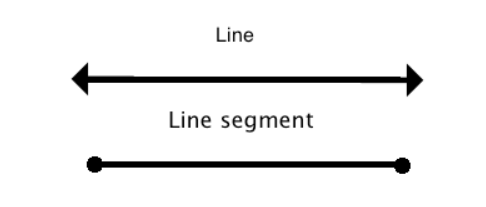A ray is defined as a mix between a line and a line segment. It extends to infinity in one direction, but there is an endpoint on the other end.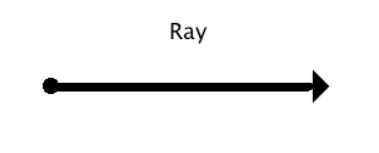Congruent line segments are defined as line segments which are equal in length. The meaning of congruent is equal. Congruent line segments are denoted by drawing the same amount of little ‘tic’ lines in the middle of the segments, and they are perpendicular to the segments. Congruent lines neither have to be parallel to each other or they have to be perpendicular to each other. They can be angled in relation to each other in various ways, the main point is that they are equal or congruent.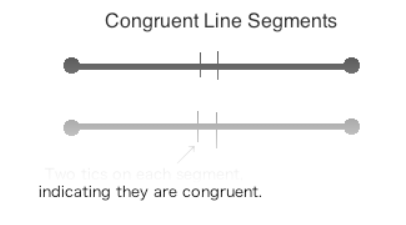## Indicating congruent line segments in writing

A line segment is indicated by drawing a line over its two end points.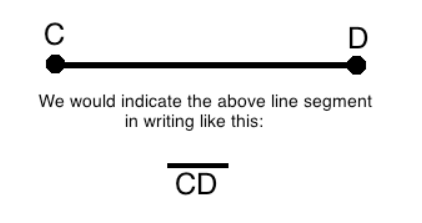Two lines segments which are congruent to each other are indicated by using the congruence symbol.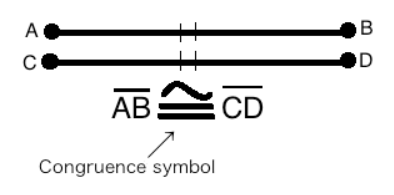Line segments are said to be congruent if they are of the same length. They are not required to be parallel to each other. They can appear at any angle or orientation on the plane. In the below given figure, there are two congruent lines which are lying at different angles.

For line segments, congruent is used for saying equals. One can say that the length of the line AB is equal to the length of line PQ. But in geometry, the correct way to express it is to say that ‘line segment AB and PQ are congruent’ or ‘AB is congruent to PQ’.

It is to be noted that rays and lines cannot be congruent because they do not have both end points defined. It extends out to infinity. So, they have no definite length.

## Symbols

The symbol for congruence is denoted as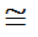.

The symbol for a line segment is denoted by a bar over two letters. So, the statements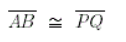can be expressed as ‘the line segments AB is congruent to the line segment PQ’.